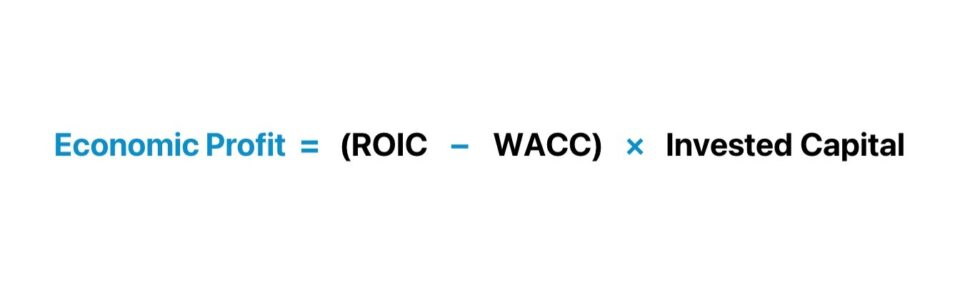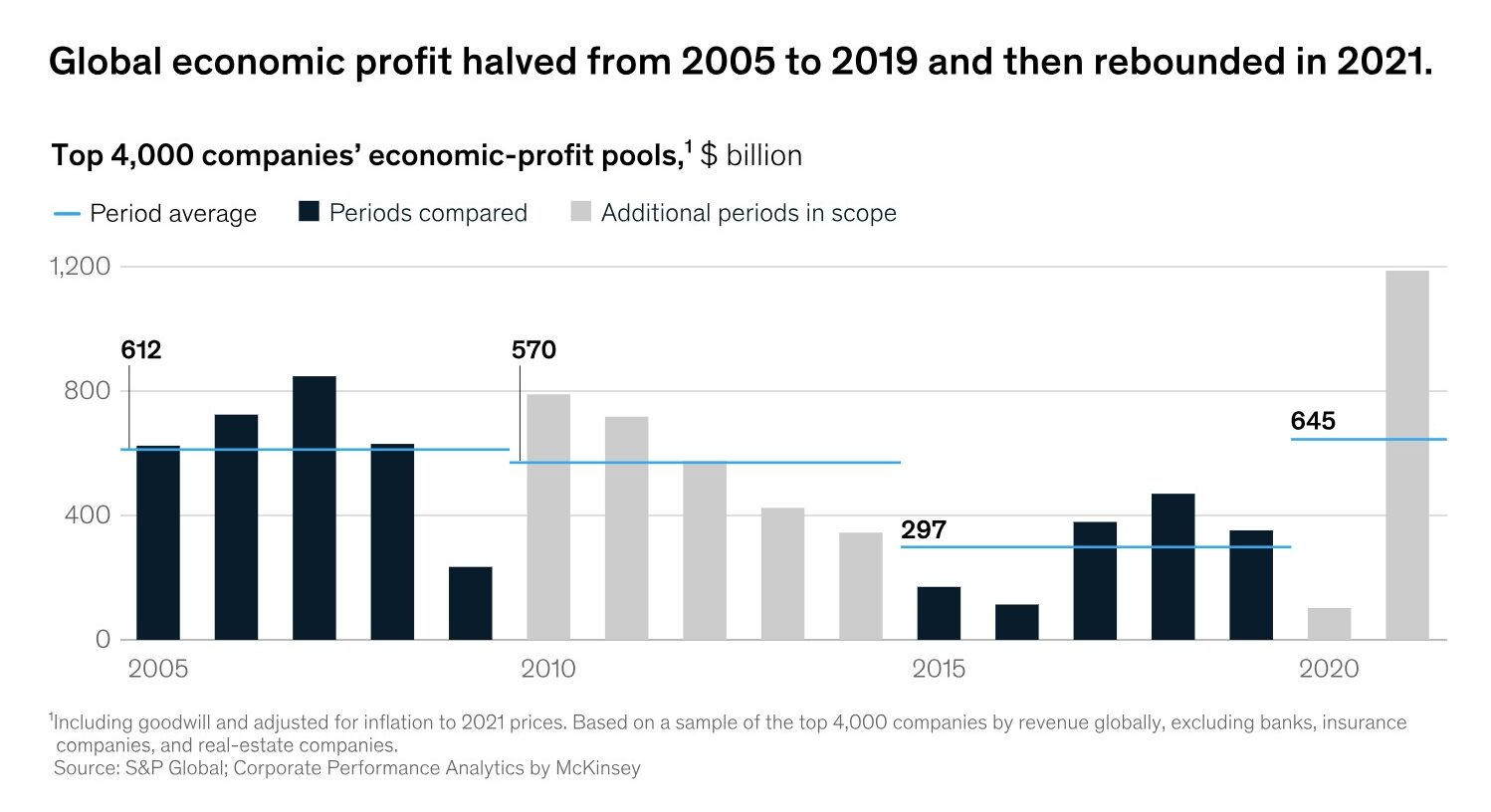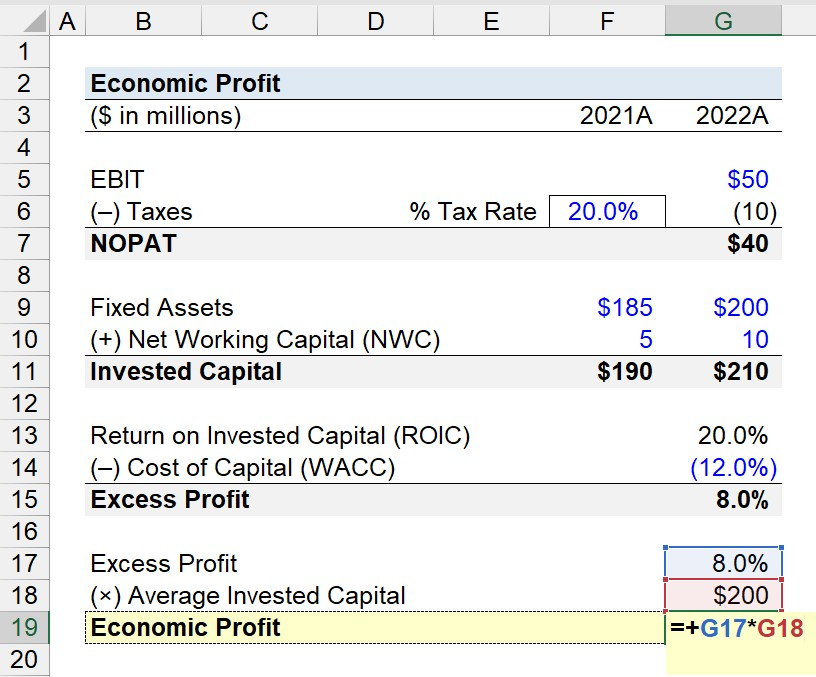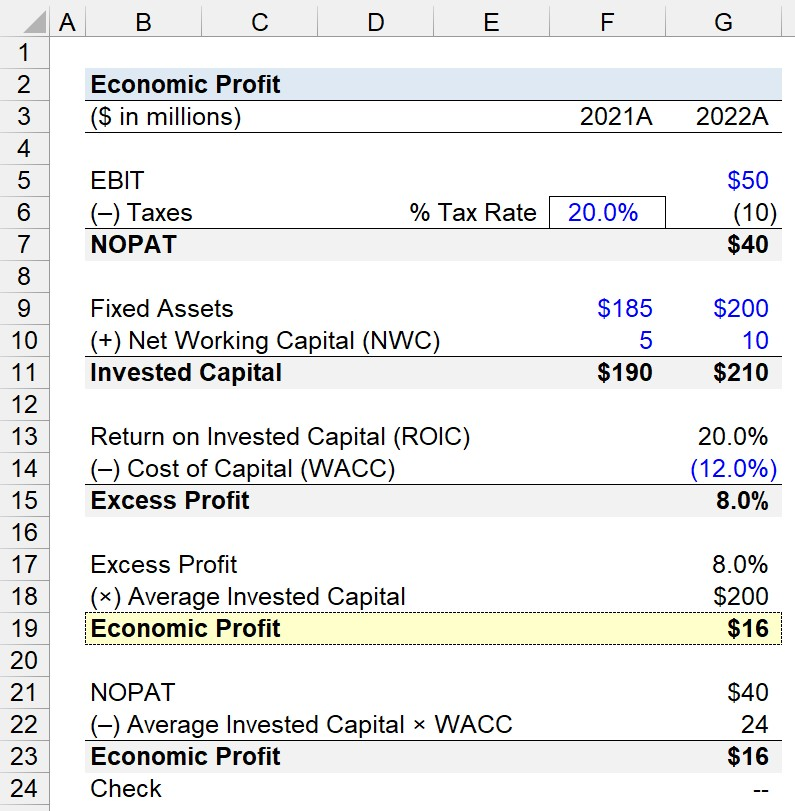Welcome to Wall Street Prep! Use code at checkout for 15% off.# Economic Profit

Guide to Understanding Economic Profit• The economic profit is the excess earnings generated by a company above the cost of capital (WACC).
• The economic profit is calculated by subtracting WACC from ROIC, and then multiplying the excess profit by the average invested capital.
• The more “residual” income produced by the company, the more economic value generated beyond its cost of capital (WACC).
• If the return on invested capital (ROIC) exceeds the cost of capital (WACC), the economic profit will be positive with positive shareholder value creation, and vice versa.

## How to Calculate Economic Profit?

Economic value is created if the residual income of a company exceeds its cost of capital, contributing to positive value creation for shareholders.

In corporate finance, value creation stems from earning a return on invested capital (ROIC) in excess of the opportunity cost of capital.

The return on invested capital (ROIC) and cost of capital (WACC) are each fundamental drivers of value creation.

• Positive Value Creation → If the return on invested capital (ROIC) exceeds the weighted average cost of capital (WACC), the economic profit will be positive.
• Negative Value Creation → If the return on invested capital (ROIC) is less than the weighted average cost of capital (WACC), the economic profit will be negative.

If the ROIC is less than the cost of capital, there is no value creation, irrespective of the growth profile of the underlying company.

Therefore, maximizing economic profit, rather than ROIC by itself, contributes to real shareholder value creation over the long run.

Companies with an ROIC consistently above their cost of capital are implied to allocate capital efficiently into profitable projects, contributing to the creation of an economic moat that protects long-term profits and market share.

The process of calculating the economic profit comprises two steps:

1. Calculate the Percent Difference between ROIC and WACC
2. Multiply the “Excess” Spread by Invested Capital

## Economic Profit Formula

The economic value can be calculated using two methods.

Economic Profit = (ROIC  WACC) × Average Invested Capital
Economic Profit = NOPAT  (Average Invested Capital × WACC)

Where:

Since the cost of capital (WACC) and NOPAT are each unlevered metrics – i.e. capital structure neutral – the economic value is the “excess” above the required rate of return attributable to the company’s capital providers, inclusive of equity shareholders and debt lenders.

###### McKinsey & Company – Shrinking Global Economic-Profit Pools

“The wider the spread between a company’s ROIC and its cost of capital, the more economic profit its capital will create.”(Source: McKinsey & Company)

## Economic Profit Calculator

We’ll now move to a modeling exercise, which you can access by filling out the form below.#### Excel TemplateSubmitting...

## Economic Profit Calculation Example

Suppose we’re tasked with calculating the economic profit of a company for fiscal year 2022.

In 2022, the company generated \$50 million in operating income (EBIT), which we’ll tax-affect using a tax rate of 20.0% to determine NOPAT.

• EBIT = \$50 million
• Taxes = (20.0%) × \$50 million = (\$10 million)
• NOPAT = \$50 million + (\$10 million) = \$40 million

On the balance sheet, the company had \$185 million and \$200 million in fixed assets for fiscal years 2021 and 2022, with \$5 million and \$10 million in net working capital (NWC).

The invested capital for each year equals the sum of the two line items, which is \$190 million and \$210 million, respectively.

• Invested Capital, 2021A = \$185 million + \$5 million = \$190 million
• Invested Capital, 2022A = \$200 million + \$10 million = \$210 million
• Average Invested Capital = (\$190 million + \$210 million) ÷ 2 = \$200 million

Since NOPAT was \$40 million in 2022, we’ll divide by the average invested capital (\$200 million) to determine the return on invested capital (ROIC) as 20.0%.

• Return on Invested Capital (ROIC) = \$40 million ÷ \$200 million = 20.0%

For the cost of capital (WACC) input, we’ll assume the company’s WACC is 12.0%, which we’ll deduct from its ROIC, resulting in an excess profit of 8.0%.

• Excess Profit = 20.0% + (12.0%) = 8.0%

The excess profit, expressed in percentage form, is multiplied by the average invested capital to obtain \$16 million for the economic profit.

• Economic Profit = 8.0% × \$200 million = \$16 millionIn conclusion, our final step is to confirm the accuracy of our work by subtracting the product of the company’s average invested capital and WACC from its NOPAT to arrive at \$16 million, which matches our prior calculation.

• Economic Profit = \$40 million – (\$200 million × 12.0%) = \$16 millionStep-by-Step Online Course

### Everything You Need To Master Financial Modeling

Enroll in The Premium Package: Learn Financial Statement Modeling, DCF, M&A, LBO and Comps. The same training program used at top investment banks.